# Math in Focus Grade 4 Chapter 6 Practice 2 Answer Key Subtracting Fractions

Practice the problems of Math in Focus Grade 4 Workbook Answer Key Chapter 6 Practice 2 Subtracting Fractions to score better marks in the exam.

## Math in Focus Grade 4 Chapter 6 Practice 2 Answer Key Subtracting Fractions

Find the equivalent fraction. Complete the model. Then subtract.

Example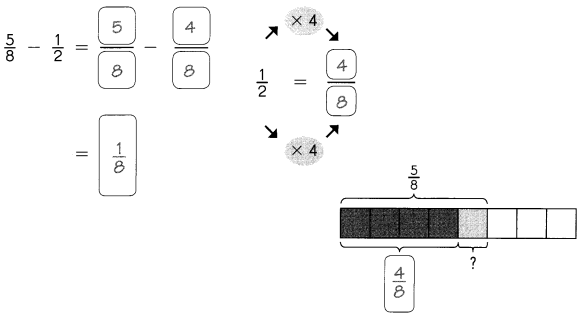Question 1.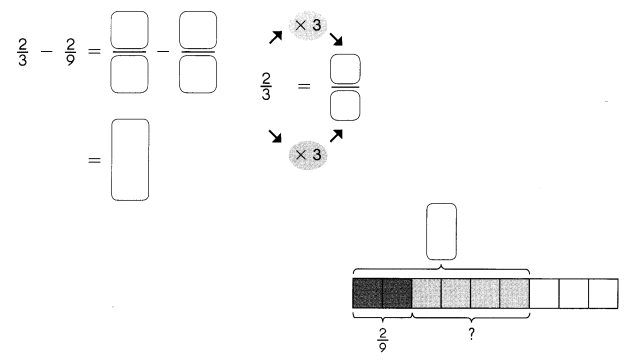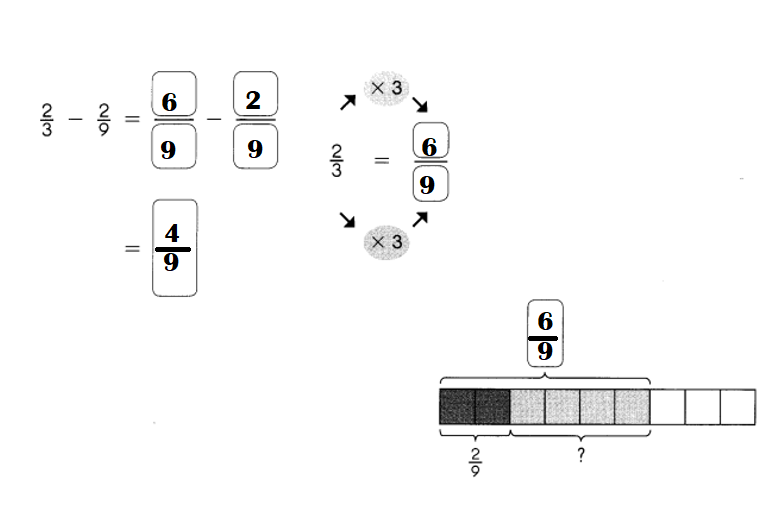Explanation:
$$\frac{2}{3}$$ – $$\frac{2}{9}$$
= (6 – 2) ÷ 9
= 4 ÷ 9 or $$\frac{4}{9}$$

Subtract. Write each answer in simplest form.
Question 2.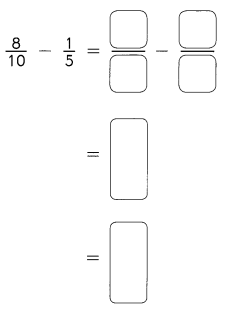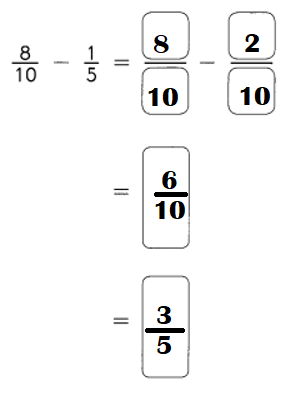Explanation:
$$\frac{8}{10}$$ – $$\frac{1}{5}$$
= (8 – 2) ÷ 10
= 6 ÷ 10
= 3 ÷ 5 or $$\frac{3}{5}$$

Question 3.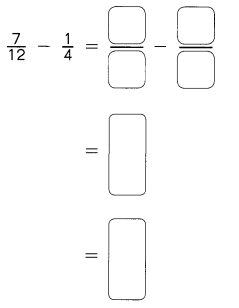Explanation:
$$\frac{7}{12}$$ – $$\frac{1}{4}$$
= (7 – 3) ÷ 12
= 4 ÷ 12
= 1 ÷ 3 or $$\frac{1}{3}$$

Question 4.
The difference between $$\frac{7}{8}$$ and $$\frac{1}{4}$$ is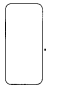The difference between $$\frac{7}{8}$$ and $$\frac{1}{4}$$ is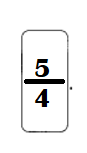Explanation:
Difference:
$$\frac{7}{8}$$ – $$\frac{1}{4}$$
= (7 – 2) ÷ 4
= 5 ÷ 4 or $$\frac{5}{4}$$

Question 5.
The difference between $$\frac{7}{12}$$ and $$\frac{1}{3}$$ isThe difference between $$\frac{7}{12}$$ and $$\frac{1}{3}$$ is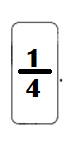$$\frac{7}{12}$$ – $$\frac{1}{3}$$
= 1 ÷ 4 or $$\frac{1}{4}$$